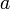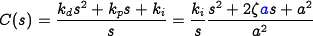# Errata: In equation (12.16), a is not defined and a factor of a is missing in numerator the last term

The parameterin this example is the same as the natural frequencyin Example 9.9 (the change in notation is needed later in the problem). In addition, the right hand side of the expression for the controller transfer function is missing a factor of: